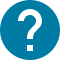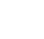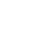学部後期課程
HOME 学部後期課程 数値シミュレーション技法

UTASにアクセスできない方は、担当教員または部局教務へお問い合わせ下さい。

# 数値シミュレーション技法

Mathematical modeling
In this course, students will learn the fundamentals of mathematical modeling. A model is a miniature representation of the real world. Mathematical modeling is a process of developing models in mathematical terms. It is an abstraction of the real world to help us understand its essence clearly by simplifying its complexity. It also helps to formulate various problems concerning our lives for finding their solutions. The concept of mathematical modeling is a common basis for virtually all empirical sciences—not only natural and engineering sciences but also human sciences such as economics, psychology, sociology, etc.

The lectures introduce three types of mathematical models: optimization models, dynamic models, and probability models. Part I begins with concepts of mathematical optimization, then moves on to the calculus for finding optimal solutions, focusing on computational techniques. Part II explores the behavior of both discrete and continuous dynamical systems. The fundamental concepts include state space and state variables. Analytic methods as well as simulation techniques for analysis of dynamical systems are presented. Part III deals with modeling techniques for probabilistic phenomena and stochastic processes. It overviews some of the key concepts of probability and statistics and also discusses some analytical techniques such as Markov chains and Monte Carlo simulation.

In each part, the students are given opportunities to work on computer programming and numerical algorithms by the use of software utilities in practice sessions.

コース名

08D14201
FAS-DA4F20L3

S2

2

NO

Schedule: The class will basically meet on Thursday 2nd and 3rd Periods of S2 Term. The detailed schedules are the following: June 8, 2023: 2nd and 3rd Periods (Sessions 0-2) June 15, 2023: 2nd and 3rd Periods (Sessions 3-4) June 22, 2023: 2nd and 3rd Periods (Sessions 5-6) June 29, 2023: 2nd and 3rd Periods (Sessions 7-8) July 6, 2023: 2nd and 3rd Periods (Sessions 9-10) July 13, 2023: 2nd and 3rd Periods (Sessions 11-12) (Note that in most days, 3rd Period sessions are planned to be self-study style practice sessions as indicated below in the outline, but they are subject to change. For those who may have time conflict on these 3rd Period sessions, flexible arrangement will be possible.) The room for the class is Komaba Bldg.8 Room 8-207. Outline First Day: Introduction 0. Outline of the course Part I: Optimization models 1. Optimization concepts 2. Practice session 1 3. Computational methods for optimization 4. Practice session 2 Part II: Dynamic models 5. Dynamical systems and analysis 6. Practice session 3 7. Simulation methods 8. Practice session 4 Part III: Probability models 9. Probability and stochastic processes 10. Practice session 5 11. Monte Carlo simulation 12. Practice session 6 Review Sessions 13. Review session (by appointment)

Lecture, Computer work

Grading is based on (1) assignments that need lab work (60%) and (2) class attendance (40%). No final examination.

Meerschaert, Mark M. Mathematical Modeling, Fourth Edition. Elsevier Inc., 2013.

Bender, Edward A. An Introduction to Mathematical Modeling. Dover Publications, 2000.

(1) Junior Division math Foundation Courses are prerequisite.
その他
Contact info: Akira Maeda: *****